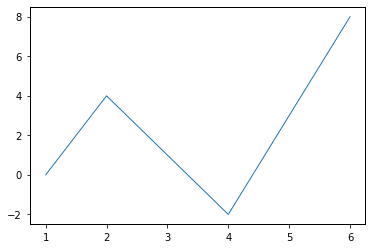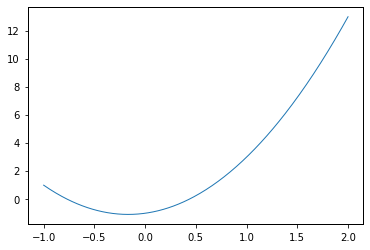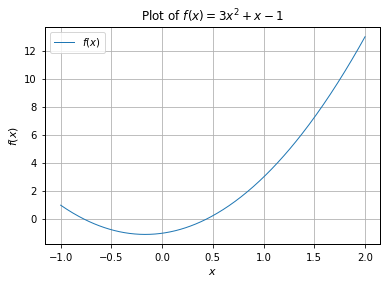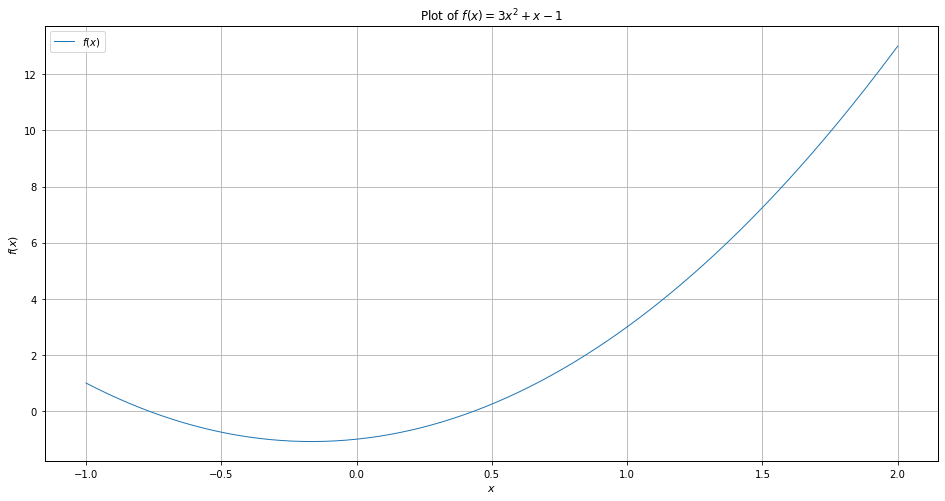# Basic Plotting in Python using Matplotlib¶

### Modules - Basics¶

Last edited: October 15th 2019

The following is a brief introduction to plotting in Python, using the library matplotlib. From the matplotlib home page: "matplotlib tries to make easy things easy and hard things possible." Let's get started!

In :
import matplotlib.pyplot as plt
# This command imports the matplotlib-package pyplot, and defines plt as an alias for pyplot
import numpy as np
# This imports the the NumPy (Numerical Python) package, aliased as np.


Above we have imported pyplot (a matplotlib package containing all useful functions for plotting), and numpy, a package for scientific computing with Python. You can learn more about numpy in this notebook. Also, notice how we have given shorter nicknames to both the numpy and pyplot import, in order to save some typing.

### The very basics of plotting¶

We use the function plt.plot to plot. This command takes two arguments: a list of $x$-values and a list of $y$-values. Each pair of $x$- and $y$-values will be a point, and a line will be drawn between these points.

In :
# Plot some arbitrary x- and y-values
x = [1, 2, 4, 6]  # List of x-values
y = [0, 4, -2, 8] # List of corresponding y-values
plt.plot(x, y)    # Function to plot the x and y points
plt.show()        # Not necessary in notebook, but required if running python from command line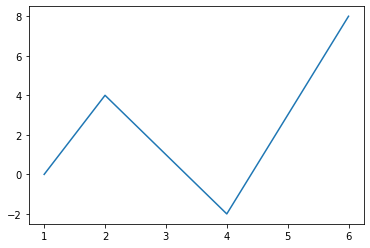### Plotting a Function¶

Plotting a function $f$, given $$y=f(x)$$ along an interval $$x=[a,b].$$

Example:

We would like to plot the function $$f(x)=3x^2+x-1$$ for the interval $$x=[-1,2].$$

Step 1: Defining the range of $x$

We use the command

In :
x = np.linspace(-1, 2, 301)  # Array of 301 linearly spaced points between -1 and 2


This creates an array of $301$ linearly spaced, discrete values of x between $-1$ and $2$. Here we used a function from the numpy library. If you are unfamiliar with numpy, and want to learn more about it, see [this] notebook.

Step 2: Using the plot command

As above, we use the plt.plot to plot the function.

In our case, we need to write

In :
plt.plot(x, 3*x**2+x-1)  # Remember, first argument is x-values, second is y-values
plt.show()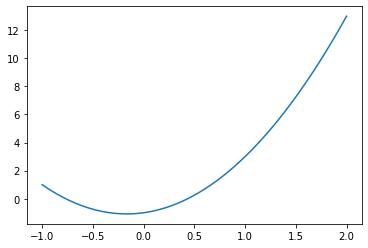Further Steps: Adding axes labels, a legend, a plot title, etc.

We often want to add axes labels, a legend, a title, a grid etc. to the plot. This is done by using the following commands:

In :
plt.plot(x, 3*x**2+x-1, label="$f(x)$") # Label is the text that will appear in the legend
plt.ylabel('$f(x)$')                    # Label on y-axis.
# The dollar symbols means to use LaTeX
plt.xlabel('$x$')                       # Label on x-axis
plt.title('Plot of $f(x)=3x^2+x-1$')    # Plot title
plt.show()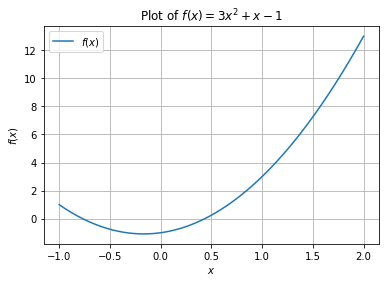Comment and uncomment the various commands to see how they effect the plot. Also notice how we used $\LaTeX$ syntax for math in labels and title by encapsulating the text in $-signs. You can also change the size of the figure quickly by using the following command: In : plt.figure(figsize=(16, 8)) # Create figure and change size # The same as previous example plt.plot(x, 3*x**2+x-1, label="$f(x)$") plt.ylabel('$f(x)$') # Label on y-axis plt.xlabel('$x$') # Label on x-axis plt.title('Plot of$f(x)=3x^2+x-1$') # Plot title plt.legend() # Add legend plt.grid() # Grid plt.show()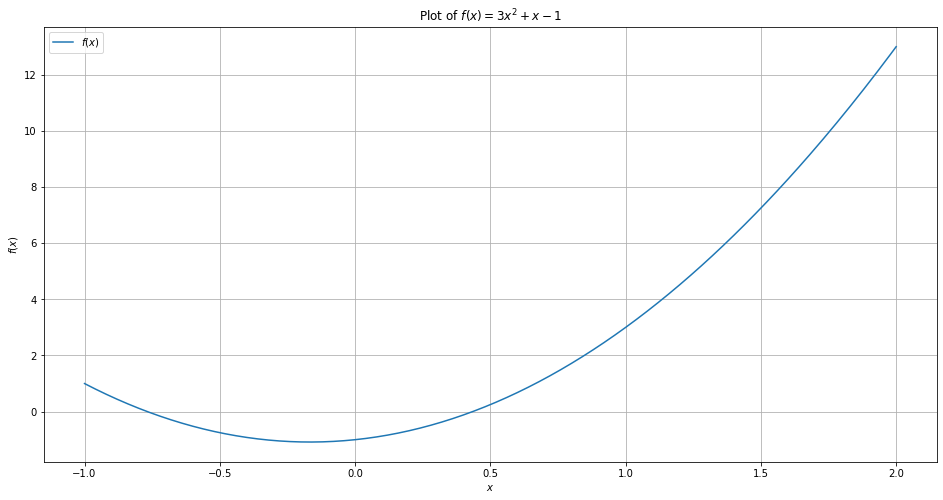The plt.figure() command also lets you set other parameters related to the figure appearance. In most of the other modules and examples at NumFys, we have set common figure parameters in the beginning of the module/example, by using the following lines of code: In : # Set figure parameters for all plots newparams = {'axes.labelsize': 11, 'axes.linewidth': 1, 'savefig.dpi': 300, 'lines.linewidth': 1.0, 'figure.figsize': (16, 8), 'ytick.labelsize': 10, 'xtick.labelsize': 10, 'ytick.major.pad': 5, 'xtick.major.pad': 5,} plt.rcParams.update(newparams)  ### Multiple Functions in One Plot¶ Let us plot $$g(x)=3x^3+1$$ in addition to our previous function: In : plt.plot(x, 3*x**2+x-1, label="$f(x)$") plt.plot(x, 3*x**3+1, label="$g(x)$") # Create legend plt.legend() plt.show()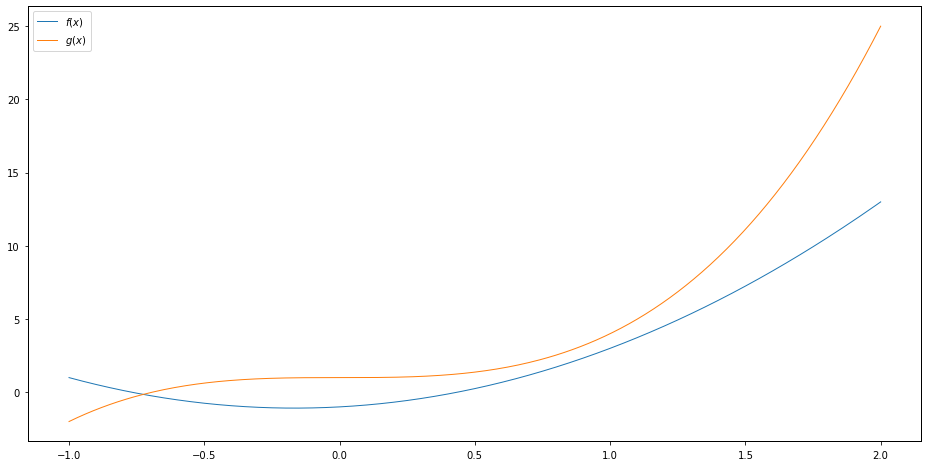## Final Note¶ There are many more commands and options for plotting functions than presented in this module. However, we covered the most important ones. We advice you to use the matplotlib and pyplot online references when additional plotting features are required. ## All code¶ The entire code in this notebook: In : # You can ignore this cell, # it only resets the changes from the code above plt.rcParams.update({'figure.figsize': (6, 4)})  In : import matplotlib.pyplot as plt # This command imports the matplotlib-package pyplot, and defines plt as an alias for pyplot import numpy as np # This imports the the NumPy (Numerical Python) package, aliased as np. # Plot some arbitrary x- and y-values x = [1, 2, 4, 6] # List of x-values y = [0, 4, -2, 8] # List of corresponding y-values plt.plot(x, y) # Function to plot the x and y points plt.show() # Not necessary in notebook, but required if running python from command line x = np.linspace(-1, 2, 301) # Array of 301 linearly spaced points between -1 and 2 plt.plot(x, 3*x**2+x-1) # Remember, first argument is x-values, second is y-values plt.show() plt.plot(x, 3*x**2+x-1, label="$f(x)$") # Label is the text that will appear in the legend plt.ylabel('$f(x)$') # Label on y-axis. # The dollar symbols means to use LaTeX plt.title('Plot of$f(x)=3x^2+x-1$') # Plot title plt.legend() # Add legend plt.grid() # Add grid plt.show() plt.figure(figsize=(16, 8)) # Create figure and change size # The same as previous example plt.plot(x, 3*x**2+x-1, label="$f(x)$") plt.ylabel('$f(x)$') # Label on y-axis plt.xlabel('$x$') # Label on x-axis plt.title('Plot of$f(x)=3x^2+x-1$') # Plot title plt.legend() # Add legend plt.grid() # Grid plt.show() # Set figure parameters for all plots newparams = {'axes.labelsize': 11, 'axes.linewidth': 1, 'savefig.dpi': 300, 'lines.linewidth': 1.0, 'figure.figsize': (16, 8), 'ytick.labelsize': 10, 'xtick.labelsize': 10, 'ytick.major.pad': 5, 'xtick.major.pad': 5,} plt.rcParams.update(newparams) plt.plot(x, 3*x**2+x-1, label="$f(x)$") plt.plot(x, 3*x**3+1, label="$g(x)\$")
# Create legend
plt.legend()
plt.show()